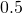# Sine Function Introduction

The word sine comes from a mistranslation of the Sanscrit word for Half Chord.

In the circle above (which has radius 1), we see a half chord, drawn from point A to the x axis. As we change the value of the angle alpha, the length of this half chord changes. The study of the lengths of these half chords is the origin of the sine function.

Notice that in the first quadrant and second quadrants, the length of the half chord is equal to the y coordinate of the point A. We define the sine of angle alpha to be the y coordinate of the point A.

When, we see that the y coordinate of point A is. We say,.

The graph on the right has point C marked at.

Move the slider to see how the sine (the y coordinate of point A) changes as the angle changes.

## Comprehension Exercises:

You may use the applet above to help answer these questions.

1. What is the value of sin(122°)?
2. What is the value of sin(256°), correct to two decimal places?
3. Is the sine of 272° positive or negative?4. In which quadrant is the angle 95°?
5. In which two quadrants is the value of sin(α°) negative?